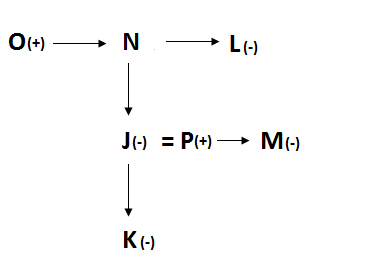# “20-20” Reasoning Questions | Crack SBI Clerk 2018 Day-122

Dear Friends, Find the list of important Reasoning Test Questions 20-20 for upcoming SBI Clerk and competitive exams. We regularly provide 20 reasoning test questions daily for students. Aspirants practice these questions on a regular basis to improve your score in reasoning section. Aspirants preparing for the exams can make use of this 20-20 Reasoning Questions. SBI Clerk 2018 Notification has been released we hope you all have started your preparation. Here we have started New Series of Practice Materials specially for SBI Clerk 2018. Aspirants those who are preparing for the exams can use this “20-20” Reasoning Questions.

[WpProQuiz 2590]

Click “Start Quiz” to attend these Questions and view Explanation

Direction (1-5): Read the given information carefully and answer the questions given below.

Seven people Jana, Tamu, Somu, Sita, Banu, Ramu and Kala are graduated in seven different streams namely BBA, BE, BCA, BE, B.sc, B.Ed and B.Com. They are all going to tour in seven different days of a week starting from Monday to Sunday. All the given information is not necessary to be in the same order.

1). Who among the following is a BBA graduate?

a). Jana

b). Banu

c). Ramu

d). Kala

e). None of these

2). Which of the following Graduate goes to tour on Thursday?

a).B.Com

b). BBA

c). BCA

d). B.Ed

e). None of these

3).Who among the following is going to tour on Tuesday?

a). Jana

b). Banu

c). Ramu

d). Kala

e). None of these

4).How many persons are going to tour between B.com graduate and Somu?

a). One

b). Two

c). Three

d). More than Three

e). None

5). How many people are going to tour before Tamu?

a). One

b). Two

c). Three

d). More than Three

e). None

Direction (6-10): Read the given information carefully and answer the questions given below.

Eight people A, B, C, D, E, F, G and H are sitting in a linear table all of them were facing towards East but not necessary to be in the same order.

A sits second to the left of G, who is an immediate neighbour of D. Only two person sits between G and H, either of them sits at extreme ends of the table. Only one person sits between D and C. B sits to the immediate left of E. F is an immediate neighbour of H and does not sits at extreme ends of the table. D is not an immediate neighbour of F.

6). Who among the following sits exactly between F and A?

a). E

b). D

c). C

d). G

e). None of these

7). Who sits third to the left of G?

a). E

b). D

c). C

d). G

e). None of these

8). How many person sits between H and D?

a). One

b). Two

c). Three

d). More than Three

e). None

9). Who sits third to the right of B?

a). E

b). D

c). C

d). G

e). None of these

10). If all the person are made to sits in alphabetical order from top to bottom, then how many of them remains in their original position?

a). One

b). Two

c). Three

d). More than Three

e). None

Direction (11-15): Read the given information carefully and answer the questions given below.

A \$ B’ means ‘A is greater than B’

A & B’ means ‘A is greater than or equal to B’

A @ B’ means ‘A is less than B’

A ! B’ means ‘A is less than or equal to B’

A % B’ means ‘A is equal to B’

a). If only conclusion I follows

b). If only Conclusion II follows

c). If either conclusion I or Conclusion II follows

d). If neither conclusion I nor Conclusion II follows

e). If both Conclusion I and Conclusion II follows

11).

Statement: G % H @ S ! A & Z \$ C @ B ! M; P % L \$ A @ R & E

Conclusion:

I). R \$ C

II). G @ P

12).

Statement: L @ J % K ! H & G \$ D; R & T @ K \$ P & O

Conclusion:

I). O % L

II). H \$ T

13).

Statement: A \$ S % F & Y @ D & C ! V; Q @ W ! Y % X \$ Z

Conclusion:

I). Q @ D

II). Z ! A

14).

Statement: M & N @ C ! T \$ E % R; P % O ! M & B @ J ! Q

Conclusion:

I). B @ N

II).N % B

15).

Statement: M & L % K ! J \$ H @ N ! B; P & O \$ K % W \$ Q

Conclusion:

I). J & Q

II). B ! O

Direction (16-18): Read the given information carefully and answer the questions given below.

P stands 3 meter north of Q. R stands 2 meter west of Q. T stands 1 meter east of S. R stands 2 meter south of S. V stands 4 meter north of W. T stands 2 meter south of U, who stands 3 meter west of V.

16). On what direction does P stands with respect to V?

a). North West

b). South West

c). North East

d). South East

e). None of these

17). What is the distance between Q and W?

a). 3 meters

b). 4 meters

c). 2 meters

d). Cannot be determined

e). None of these

18). What is the shortest distance between T and W?

a). √13

b). √17

c). √19

d). Cannot be determined

e). None of these

Direction (19-20): Read the given information carefully and answer the questions given below.

J, K, L, M, N, O and P are the seven people in a family all are inter related to each other. There is one married couple and three generations in the family. J is mother of K, who is the only granddaughter of N. N has only one brother. M is the sister of P. O is brother of L, whose only niece is J. P is husband of J.

19). How is P related to L?

a). Son

b). Son-in-law

c). Nephew

d). Uncle

e). Cannot be determined

20). If L has only one brother, Then how is N related to K?

a). Grand Mother

b). Grand Father

c). Grand Son

d). Grand Daughter

e). None of these

Direction (1-5):

Explanation: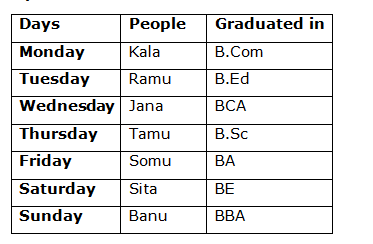i). The person is going to tour on Wednesday is a BCA graduate.

ii). Sita is an BE graduate and she is going to tour on Saturday.

iii). Only one person is going to tour between B.Ed graduate and B.Sc graduate.

iv). B.Com graduate is going to tour one of the day before BA graduate, who is going to tour one of the day before BBA graduate.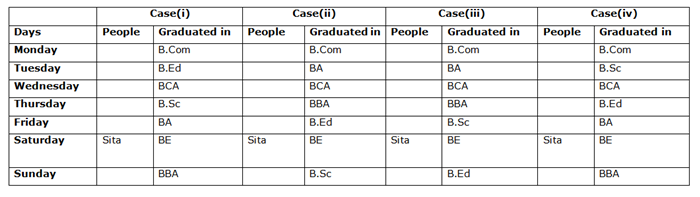v). Only one person is going to tour between BBA graduate and Somu.

vi). Kala is going to tour on Monday.

vii). Only one person is going to tour between B.Ed graduate and Tamu, who is not a BCA graduate.

ix). Ramu is going to tour immediately before Jana.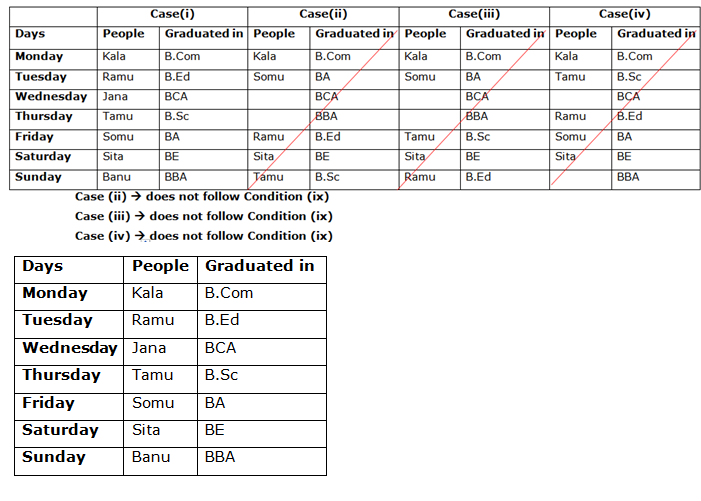Direction (6-10):

Explanation: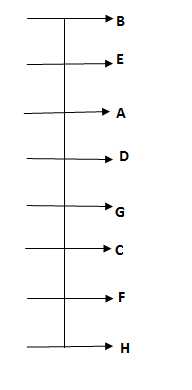i). A sits second to the left of G, who is an immediate neighbour of D.

ii). Only two person sits between G and H, either of them sits at extreme end of the table.

iii). Only one person sits between D and C.

iv). B sits immediate left of E.

v). F is an immediate neighbour of H and does not sits at extreme end of the line.

vi). D is not an immediate neighbour of F.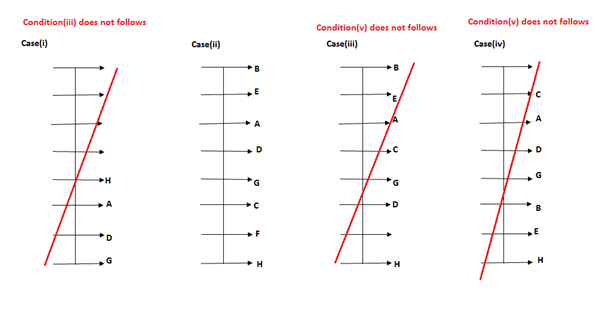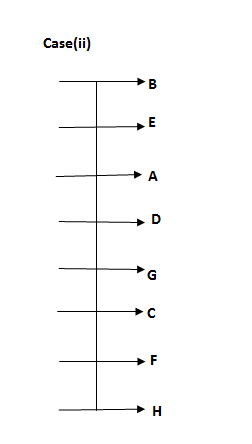Direction (11-15):

Explanation: G = H < S ≤ A ≥ Z > C < B ≤ M; P = L > A < R ≥ E

I). R > A ≥ Z > C (True)

II). G = H < S ≤ A < L = P (True)

Explanation: L < J = K ≤ H ≥ G > D; R ≥ T < K > P ≥ O

I). L < J = K > P ≥ O (False)

II). T < K ≤ H (True)

Explanation: A > S = F ≥ Y < D ≥ C ≤ V; Q < W ≤ Y = X > Z

I). Q < W ≤ Y < D (True)

II). A > S = F ≥ Y = X > Z (False)

Explanation: M ≥ N < C ≤ T > E = R; P = O ≤ M ≥ B < J ≤ Q

B ≤ M ≥ N

Explanation: M ≥ L = K ≤ J > H < N ≤ B; P ≥ O > K = W > Q

I). J ≥ K = W > Q (False)

II).  O > K ≤ J > H < N ≤ B (False)

Direction (16-18):

Explanation: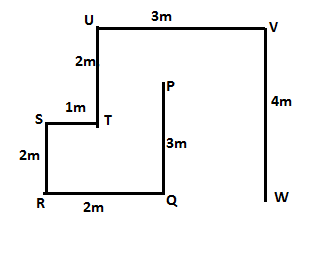Direction (19-20):

Explanation: